RS Aggarwal Class 8 Solutions Chapter 6 - Operations On Algebraic Expressions

RS Aggarwal Class 8 Chapter 6 - Operations On Algebraic Expressions Solutions Free PDF

In this chapter, the students will learn about monomials, binomials, and polynomials. In addition to these, the students will also learn the addition and subtraction of algebraic expressions. Learn the concepts of algebraic expressions by solving questions from RS Aggarwal for class 8. RS Aggarwal for class 8 has covered all the basic and advanced concepts. For solutions to these questions, students can also refer to RS Aggarwal class 8 solutions chapter 6 operations on algebraic expressions.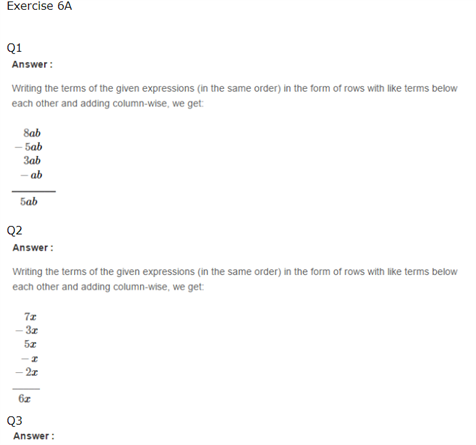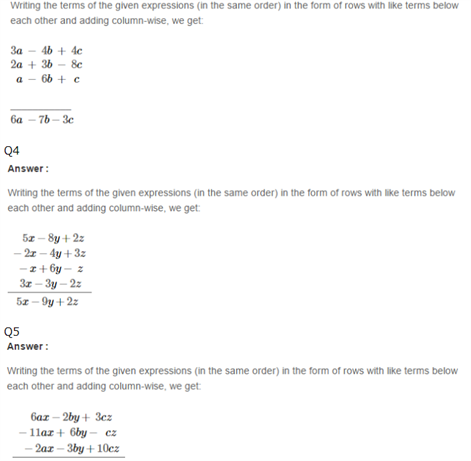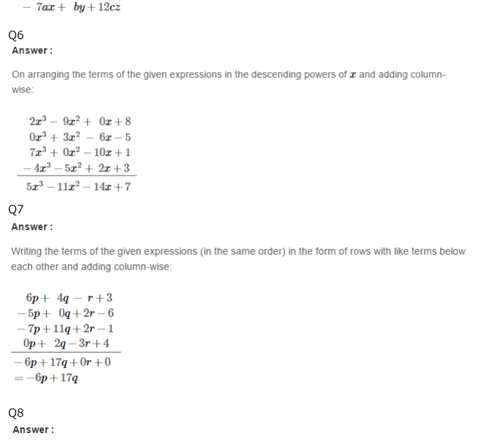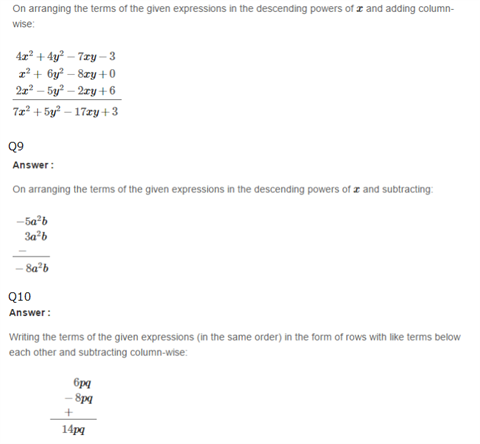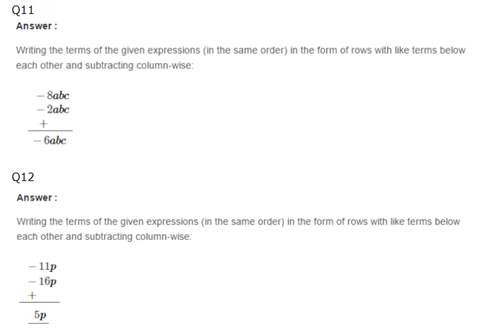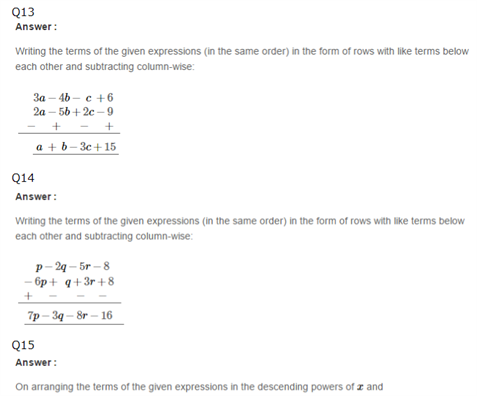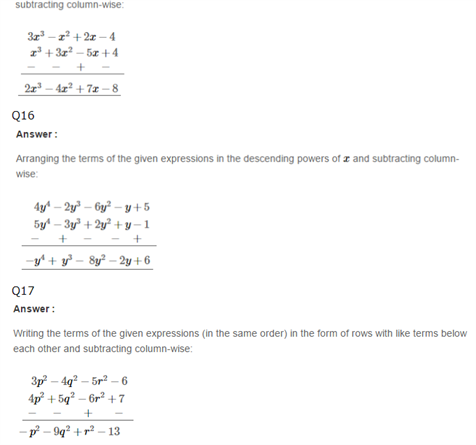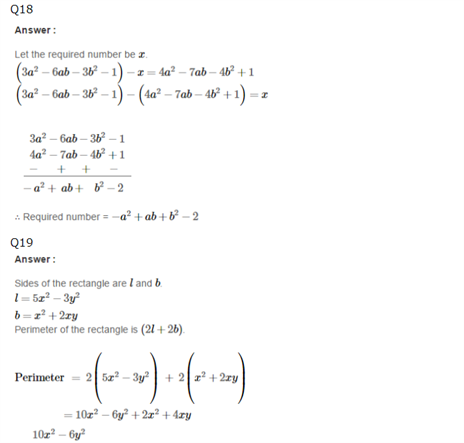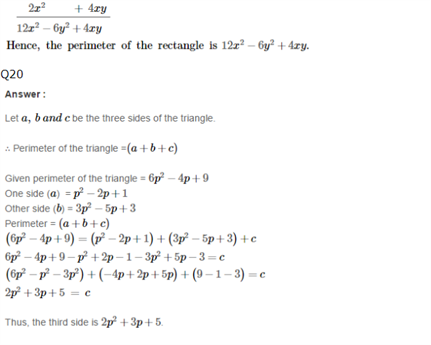Practise This Question

Which part of our body vibrates to produce sound when we speak?